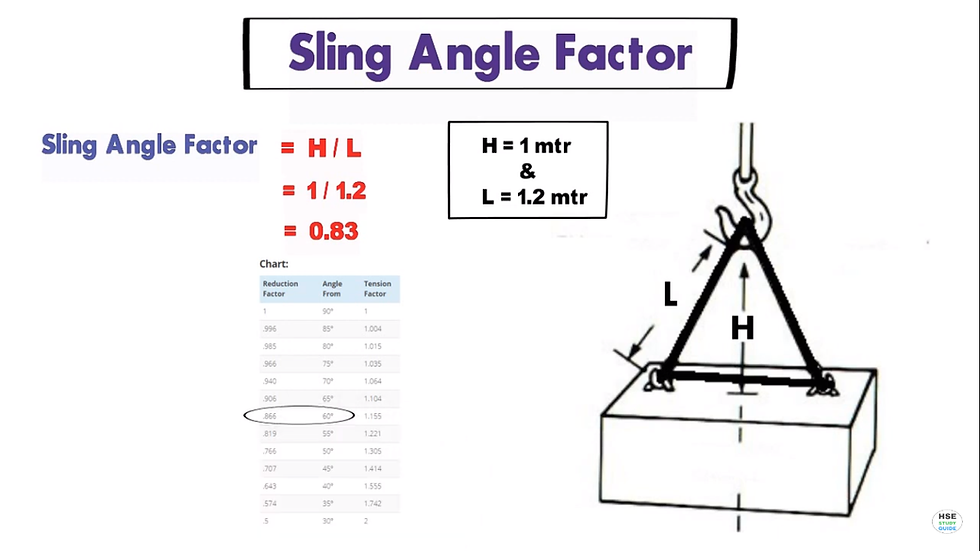top of page

# How to calculate Sling capacity and Sling angle calculation formula

Updated: May 17, 2022Sling WLL at specified angle = Sling WLL x No. of legs x Sling angle factorExample:- Sling (WLL) = 2000kg

Sling angle = 60 degree

No. of legs = 2

Sling capacity (WLL) at a 60-degree angle?

Now, put these value in the above formula

Sling WLL at a 60-degree angle = 2000kg x 2 x 60 degree

= 2000kg x 2 x 0.866

= 3464kg

Note:- if we consider a 60-degree angle for sling then the reduction factor is 0.866 as per the chart.Consider, we don't know the sling angle then how we can calculate the sling angle factor?

Sling angle factor = H/L ( H - Height & L- Length)

= 1/1.2

= 0.83See All
bottom of page